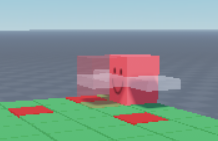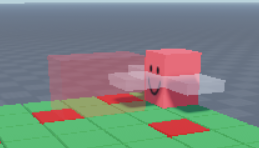# Spawn a part with various size infront of another part but the gap stay the same

I am trying to spawn a part infront of another part. The part that will spawn will have various sizes based on an intvalue. for example if I change the value to 1 then the part size would be (4, 5, (1 * 4)) if I change the value to 2 then it would be (4, 5, (2 * 4)) (I mutiply the intvalue by 4 because my game is based on 4x4 block that form into a grid) I got the size working but the problem is the gap between the spawn part and another main part, I want the gap between them to stay the same no matter how much the size of the spawn part would be. kinda like this

blue = main part
red = spawn part

When I tried it, it became like thisIntvalue = 1

The picture above is exactly what I wanted but when I change the intvalue to 2, this happensas you can see, the size changed correctly but the position is not that accurate, I want the gap between then to stay the same like in the first one, but for this one its move to the front a bit.

Here’s my script

``````script.Parent.MouseClick:Connect(function()
if deb == false then
deb = true
local RangeValue = StatsFolder.Range.Value
local Range = (RangeValue * 4)

local offset = Vector3.new(0, 0, -Range)

local Hitbox = Instance.new("Part")
Hitbox.Size = Vector3.new(4, 5, Range)
Hitbox.CFrame = Char.CFrame * CFrame.new(offset)
Hitbox.Color = Color3.fromRGB(255, 50, 50)
Hitbox.Transparency = 0.75
Hitbox.Material = "SmoothPlastic"
Hitbox.Anchored = true
Hitbox.CanCollide = false

Hitbox.Parent = game.Workspace.Hitboxes

deb = false

Hitbox:Destroy()
end
end)
``````

Anyways, if anyone got solutions please tell me in the replies, Thanks in advance!

Alright so the most important thing to keep in mind is that position is the middle of the part. This is mostly important because we need to deal with half sizes in order to keep things aligned correctly. To place a dot exactly at the end of a part you would need to do Part.Position.X + Part.Size.X / 2.

Now to refer to my beautiful 2D representation

Lets say the character is the black box, and the hitbox is the grey part. The amount we will need to move the hitbox away from the character is (Character.Size.Z / 2) + (Hitbox.Size.Z / 2).

In your current code you are simply adding the size of the hitbox directly. This results in the first one looking alright because both boxes are the same size and half plus half equals one. But when you get to the second one, you are now adding 2 more studs than you should be.

To fix this we simply need to redefine how you are settings offset.

Basically just replace this line

``````local offset = Vector3.new(0, 0, -Range)
``````

With this

``````local offset = Vector3.new(0, 0, (Range / 2) + (Char.Size.Z / 2)) * -1 --I moved the negative to the end to avoid writing extra parenthesis
``````

Though if your character will always be size 4, everything after the + with a 2 since that would always be what it returns.

1 Like